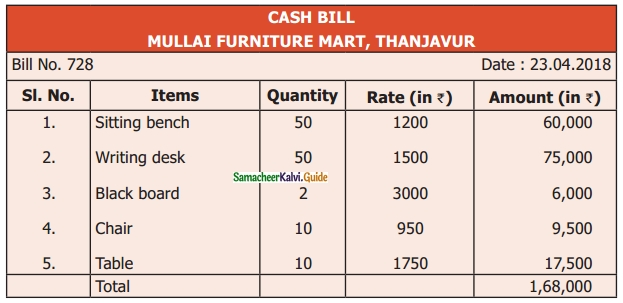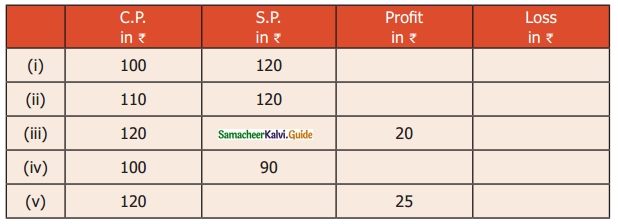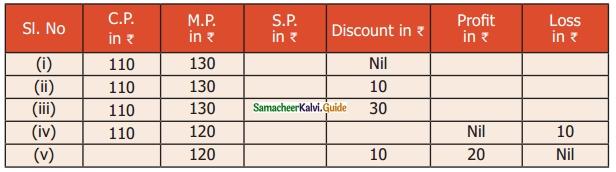Students can download Maths Chapter 3 Bill, Profit and Loss Ex 3.1 Questions and Answers, Notes, Samacheer Kalvi 6th Maths Guide Pdf helps you to revise the complete Tamilnadu State Board New Syllabus, helps students complete homework assignments and to score high marks in board exams.

## Tamilnadu Samacheer Kalvi 6th Maths Solutions Term 2 Chapter 3 Bill, Profit and Loss Ex 3.1

Question 1.
A School purchases some furniture and gets the following bill.(i) What is the name of the store?
(ii) What is the serial number of the bill?
(iii) What is the cost of a black board?
(iv) How many sets of benches and desks does the school buy?
(v) Verify whether the total bill amount is correct.
Solution:
(i) Mullai Furniture mart
(ii) Serial No :: 728
(iii) Rs 3000
(iv) 50 sets
(v) CorrectQuestion 2.
Prepare a bill for the following books of biographies purchased from Maruthu Book Store, Chidambaram on 12.04.2018 bearing the bill number 507. 10 copies of Subramanya Bharathiar @ Rs 55 each, 15 copies of Thiruvalluvar @ Rs 75 each, 12 copies of Veeramamunivar @ Rs 60 each, and 12 copies of ThiruviKa @ Rs 70 each.
Solution:Question 3.
Fill up the appropriate boxes in the following table.Solution:
(i) CP = Rs 100
SP = Rs 120
CP < SP
Profit = SP – CP
= Rs 120 – Rs 100
= Rs 20

(ii) CP = Rs 110
SP = Rs 120
CP < SP
Profit = SP – CP
= Rs 120 – Rs 110
= Rs 10(iii) CP = Rs 120
Profit = Rs 20
Profit = SP – CP
Rs 20 = SP – Rs 120
Rs 20 + Rs 120 = SP
SP = Rs 140

(iv) CP = Rs 100
SP = Rs 90
CP > SP
Loss = CP – SP
= Rs 100 – Rs 90
= Rs 10

(v) CP = Rs 120
Profit = Rs 25
Profit = SP – CP
Rs 25 = SP – Rs 120
Rs 25 + Rs 120 = SP
Rs 145 = SP
SP = Rs 145Question 4.
Fill up the appropriate boxes in the following table.Solution:
(i) CP = Rs 110
MP = Rs 130
Profit = SP – CP
= Rs 130 – Rs.110
= Rs 20
If there is no discount MP = SP
SP = Rs 130

(ii) CP = Rs 110 Profit
MP = 130
Discount = Rs 10
= SP – CP
= Rs 120 – Rs 110
= Rs 10
SP = MP – Discount
= Rs 130 – Rs 10
= Rs 120(iii) CP = Rs 110
MP = Rs 130
Discount = Rs 30
Loss = CP – SP
= Rs 110 – Rs 100
= Rs 10
SP = Mp – Discount
= Rs 130 – Rs 30
= Rs 100

(iv) CP = Rs 110
MP = Rs 120
Loss = CP – SP
SP = CP – Loss
= Rs 110 – Rs 10
= Rs 100
Discount = MP – SP
= Rs 120 – Rs 100
= Rs 20

(v) MP = Rs 120
Discount = Rs 10
Profit = Rs 20
Loss = Rs 0
SP = MP – Discount
= Rs 120 – Rs 10
= Rs 110
Profit = Rs 20
= SP – CP
Rs 20 = Rs 110 – CP
CP = SP – Profit
= Rs 110 – Rs 20
= Rs 90Question 5.
Rani bought a set of bangles for ₹ 310. Her neighbour liked it the most. So Rani sold it to her for ₹ 325. Find the profit or loss to Rani.
Solution:
CP = Rs 310
SP = Rs 325
Profit = SP – CP = Rs 325 – Rs 310 = Rs 15

Question 6.
Sugan bought a pair of jeans pant for Rs 750 not fit him. He sold it to his friend for Rs 710, Find the profit or loss to sugan.
Solution:
CP = Rs 750
SP = Rs 710
CP > SP
Loss = CP – SP
= Rs 750 – Rs 710
= Rs 40

Question 7.
Somu bought a second-hand bike for ₹ 28,000 and spent ₹ 2000 on its repair. He sold it for ₹ 30,000. Find his profit or loss. Solution:
CP = Rs 28,000 + Rs 2,000
CP = Rs 30,000
SP = Rs 30,000
CP = SP
No profit / LossQuestion 8.
Muthu has a car worth Rs 8,50,000 and he wants to sell it at a profit of Rs 25,000. What should be the selling price of the car?
Solution:
CP = Rs 8,50,000
Profit = Rs 25,000
SP = CP + Profit
= Rs 8,50,000 + Rs 25,000
= Rs 8,75,000

Question 9.
Valarmathi sold her pearl set for ₹ 30,000 at a profit of ₹ 5000. Find the cost price of the pearl set.
Solution:
SP = Rs 30,000
Profit = Rs 5,000
CP = SP – Profit
= Rs 30,000 – Rs 5,000
= Rs 25,000

Question 10.
If Guna marks his product to be sold for Rs 325 and gives a discount of Rs 30, then find the S.P.
Solution:
MP = Rs 325
Discount = Rs 30
SP = MP – Discount
= Rs 325 – Rs 30
= Rs 295Question 11.
A man buys a chair for ₹ 1500. He wants to sell it at a profit of ₹ 250 after making a discount of ₹ 100. What is the M.P of the chair?
Solution:
CP = Rs 1,500
Profit = Rs 250
SP = CP + Profit
= Rs 1,500 + Rs 250
= Rs 1,750
Discount = Rs 100
SP = MP – Discount
MP = SP + Discount
= Rs 1,750 + Rs 100
= Rs 1,850

Question 12.
Amutha marked her home product of pickle as Rs 300 per pack. But she sold it for only Rs 275 per pack. What was the discount offered by her per pack?
Solution:
MP = Rs 300
SP = Rs 275
Discount = MP – SP
= Rs 300 – Rs 275
= Rs 25

Question 13.
Valavan bought 24 eggs for ₹ 96. Four of them were broken and also he had a loss of ₹ 36 on selling them. What is the selling price of one egg?
Solution:
Cost of 24 eggs = Rs 96
Since 4 of the eggs were broken, the number of remaining eggs = 24 – 4 = 20
Since the loss is Rs 36
The selling price of 20 eggs
SP = CP – Loss
= Rs 96 – Rs 36
= Rs 60
∴ Cost of 1 egg = Rs 60 / 20 = Rs. 3Question 14.
Mangai bought a cell phone for Rs 12,585. It fell down. She spent Rs 500 on its repair. She sold it for Rs 7,500. Find her profit or loss.
Solution:
CP = Rs 12,585 + Rs 500
= Rs 13,085
SP = Rs 7,500
CP > SP
Loss = CP – SP
= Rs 13,085 – Rs 7,500
= Rs 5,585

Objective Type Questions

Question 15.
Discount is subtracted from ______ to get S.P.
(a) M.P
(b) C.P
(c) Loss
(d) Profit
Solution:
(a) M.PQuestion 16.
Overhead expenses are always included in ………
(a) S.P
(b) C.P
(c) Profit
(d) Loss
Solution:
(b) C.P

Question 17.
There is no profit or loss when ______.
(a) C.P = S.P.
(b) C.P. > S.P
(c) C.P. < S.P
(d) M.P = Discount
Solution:
(a) cost price = selling priceQuestion 18.
Discount = M.P.
(a) Profit
(b) S.P
(c) Loss
(d) C.P
Solution:
(b) S.P